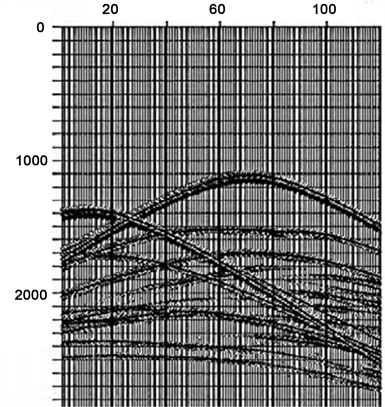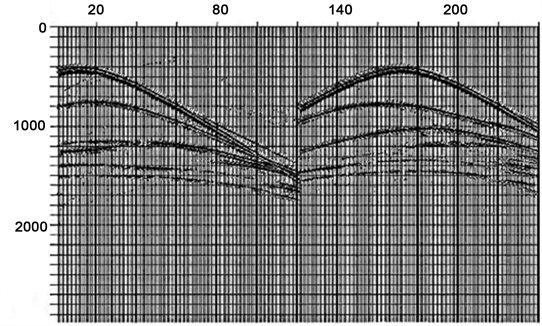﻿ 多震源盲分离技术研究

# 多震源盲分离技术研究The Research of Multi-Source Data Separation Technology

Abstract: This paper treats the separation of the double-source data as a blind signal separation problem, and introduces the basic theory of blind signal separation. In this paper, for the case where the number m of observed signals and the number n of sources are the same, a simple blind signal separation process is designed and the numerical simulation is carried out to prove the effectiveness of the proposed method.

1. 引言

2. 多震源混合信号概述

${P}_{bl}=\Gamma P$ (1)

${P}_{bl}^{j}={\sum }_{k=1}^{N}{\Gamma }_{k}^{j}{P}_{k}$ (2)

${\Gamma }_{k}^{j}$ 已知时，实际上它是一个一个线性反演问题，而当混合算子 ${\Gamma }_{k}^{j}$ 未知，则此波场分离是一个盲过程，也就是我们上面提到的BSS技术。

3. BSS简介

3.1. BSS基本原理

$\begin{array}{l}{x}_{1}\left(t\right)={a}_{11}{s}_{1}\left(t\right)+{a}_{12}{s}_{2}\left(t\right)+{a}_{13}{s}_{3}\left(t\right)\\ {x}_{2}\left(t\right)={a}_{21}{s}_{1}\left(t\right)+{a}_{22}{s}_{2}\left(t\right)+{a}_{23}{s}_{3}\left(t\right)\\ {x}_{3}\left(t\right)={a}_{31}{s}_{1}\left(t\right)+{a}_{32}{s}_{2}\left(t\right)+{a}_{33}{s}_{3}\left(t\right)\end{array}$ (3)

${x}_{i}\left(t\right)-{\sum }_{i=1}^{N}{a}_{ij}{s}_{i}\left(t\right),\text{\hspace{0.17em}}\text{\hspace{0.17em}}i=1,2,\cdots ,N$ (4)

${x}_{i}\left(t\right)-{\sum }_{i=1}^{N}{\sum }_{k=0}^{P}{a}_{ij}{s}_{i}\left(t-k\right),\text{\hspace{0.17em}}\text{\hspace{0.17em}}i=1,2,\cdots ,N$ (5)

3.2. 信号预处理

3.2.1. 去均值过程

${\stackrel{¯}{x}}_{i}\left(t\right)={x}_{i}\left(t\right)-\frac{1}{N}{\sum }_{i=1}^{N}{x}_{i}\left(t\right),\text{\hspace{0.17em}}\text{\hspace{0.17em}}i=1,2,\cdots ,N$ (6)

3.2.2. 白化过程

$\text{E}\left\{x{x}^{\text{T}}\right\}=ED{E}^{\text{T}}$ (7)

$V=E{D}^{-1/2}{E}^{\text{T}}$ (8)

$\text{E}\left\{z{z}^{\text{T}}\right\}=\stackrel{˜}{A}\text{E}\left\{s{s}^{\text{T}}\right\}{\stackrel{˜}{A}}^{\text{T}}=I$ (9)

4. 算法介绍及数据模拟

4.1. 线性混合盲分离算法

$\left\{\begin{array}{l}{y}_{1}\left(t\right)={x}_{1}\left(t\right)-{\omega }_{1}{x}_{2}\left(t\right)\\ {y}_{2}\left(t\right)={x}_{2}\left(t\right)-{\omega }_{2}{x}_{1}\left(t\right)\end{array}$ (10)

${r}_{{y}_{1}{y}_{2}}\left({t}_{1},{{t}^{\prime }}_{1}\right)=\text{E}\left[{y}_{1}\left({t}_{1}\right){y}_{2}\left({{t}^{\prime }}_{1}\right)\right]={r}_{{x}_{1}{y}_{2}}\left({t}_{1},{{t}^{\prime }}_{1}\right)-{\omega }_{1}{r}_{{x}_{2}{y}_{2}}\left({t}_{1},{{t}^{\prime }}_{1}\right)=0$ ，得到

${\omega }_{1}=\frac{{r}_{{x}_{1}{y}_{2}}\left({t}_{1},{{t}^{\prime }}_{1}\right)}{{r}_{{x}_{2}{y}_{2}}\left({t}_{1},{{t}^{\prime }}_{1}\right)}=\frac{{r}_{{x}_{1}{y}_{2}}\left({t}_{1},{\tau }_{1}\right)}{{r}_{{x}_{2}{y}_{2}}\left({t}_{1},{\tau }_{1}\right)}$ (11)

${r}_{{y}_{1}{y}_{2}}\left({t}_{2},{{t}^{\prime }}_{2}\right)=\text{E}\left[{y}_{1}\left({t}_{2}\right){y}_{2}\left({{t}^{\prime }}_{2}\right)\right]={r}_{{y}_{1}{x}_{2}}\left({t}_{2},{{t}^{\prime }}_{2}\right)-{\omega }_{1}{r}_{{y}_{1}{x}_{1}}\left({t}_{2},{{t}^{\prime }}_{2}\right)=0$ ，得到

${\omega }_{2}=\frac{{r}_{{y}_{1}{x}_{2}}\left({t}_{2},{{t}^{\prime }}_{2}\right)}{{r}_{{y}_{1}{x}_{1}}\left({t}_{2},{{t}^{\prime }}_{2}\right)}=\frac{{r}_{{y}_{1}{x}_{2}}\left({t}_{2},{\tau }_{2}\right)}{{r}_{{y}_{1}{x}_{1}}\left({t}_{2},{\tau }_{2}\right)}$ (12)

$W=\left(\begin{array}{cc}1& -{\omega }_{1}\\ -{\omega }_{2}& 1\end{array}\right)$ (13)

${W}^{\left(ij\right)}W\to W$

${W}^{\left(ij\right)}=\left[\begin{array}{cc}\mathrm{cos}{\theta }_{ij}& \mathrm{sin}{\theta }_{ij}\\ -\mathrm{sin}{\theta }_{ij}& \mathrm{cos}{\theta }_{ij}\end{array}\right]$ (14)

4.2. 算法步骤

1) 将观测得到的混合数据用X表示；

2) 求出零时间延迟相关矩阵 ${R}_{xx}\left(0\right)=E\left[X{X}^{\text{T}}\right]$

3) 对 ${R}_{xx}\left(0\right)$ 进行奇异值分解，得到 ${R}_{xx}\left(0\right)=UAU$ ，U为正交矩阵，A为对角矩阵；

4) 计算预白化矩阵 $B={A}^{-1/2}{U}^{\text{T}}$ ，则得到预白化后和混合数据为 $Z=BX;$

5) 初始化盲分离信号Y，选取一对成分 ${y}_{i},{y}_{j}$ ，计算角度 ${\theta }_{ij}$

6) 如果 ${\theta }_{ij}>\epsilon$ ，其中 $\epsilon \ll 1$ ，求出 ${W}^{\left(ij\right)}=\left[\begin{array}{cc}\mathrm{cos}{\theta }_{ij}& \mathrm{sin}{\theta }_{ij}\\ -\mathrm{sin}{\theta }_{ij}& \mathrm{cos}{\theta }_{ij}\end{array}\right]$ ，更新分离矩阵，

${W}^{\left(ij\right)}W\to W$

7) 旋转信号矩阵 $Z:Z←{W}^{\left(ij\right)}Z$ ，直到 ${\theta }_{ij}\le \epsilon$ ，否则跳回(5)。

4.3. 数值模拟Figure 1. Multi-source data (dual source)Figure 2. Separate data

5. 结语

 倪宇东. 可控震源地震勘探采集技术[M]. 北京: 石油工业出版社, 2014.

 李剑锋. 基于盲源分离的地震信号处理方法研究及应用[D]: [硕士学位论文]. 青岛: 中国石油大学(华东), 2012.

 Aapohyvarinen, Juhakarhunen, Erkk. 独立成分分析[M]. 北京: 电子工业出版社, 2014.

Top